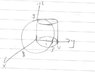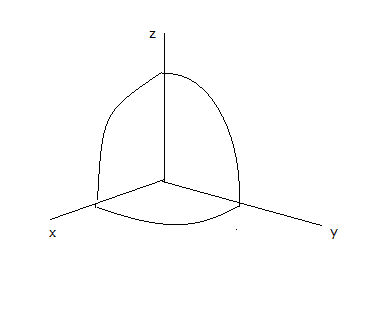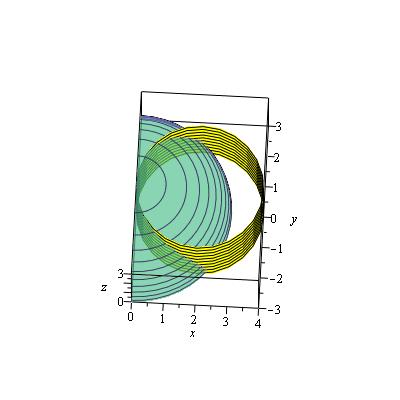# Solid bounded by different region

## Homework Statement

By using cylindrical coordinates , evaluate the volume of solid bounded on top of sphere (x^2) + (y^2) + (z^2) = 9 and it's sides by (x^2) + (y^2) = 4x . [/B]

## The Attempt at a Solution

I have sketched out the diagram , but i dun know which part is the solid formed... Can someone highlight the solid formed ? [/B]

#### Attachments

•9899.jpg
14.9 KB · Views: 350

## Homework Statement

By using cylindrical coordinates , evaluate the volume of solid bounded on top of sphere (x^2) + (y^2) + (z^2) = 9 and it's sides by (x^2) + (y^2) = 4x . [/B]

## The Attempt at a Solution

I have sketched out the diagram , but i dun know which part is the solid formed... Can someone highlight the solid formed ? [/B]

Is the wording you wrote the exact statement of the problem? If so, it is confusing, and in English may easily be misinterpreted. There is a difference in meaning between saying "bounded on top of sphere... "; the "on top" says to me that the region lies above the sphere---it is on top of the sphere. However, maybe it means "bounded on top BY the sphere..." which means the region lies below the sphere: the sphere is the top of the region. I suspect that the second interpretation is intended, but the wording of the problem could be taken to imply the opposite.

Anyway, what about a lower limit? is the opposite side of the sphere also the bottom of the region? If we don't specify a bottom, we can have ##z## extending to ##-\infty##, giving an infinite volume.

•Is the wording you wrote the exact statement of the problem? If so, it is confusing, and in English may easily be misinterpreted. There is a difference in meaning between saying "bounded on top of sphere... "; the "on top" says to me that the region lies above the sphere---it is on top of the sphere. However, maybe it means "bounded on top BY the sphere..." which means the region lies below the sphere: the sphere is the top of the region. I suspect that the second interpretation is intended, but the wording of the problem could be taken to imply the opposite.

Anyway, what about a lower limit? is the opposite side of the sphere also the bottom of the region? If we don't specify a bottom, we can have ##z## extending to ##-\infty##, giving an infinite volume.
sorry , i made a typo . The original question is evaluate the volume of solid bounded on top by the sphere (x^2) + (y^2) + (z^2) = 9...
Here's after correction , the cylinder span from z = -3 to 3 ...
What is the portion of the solid bounded on top of sphere (x^2) + (y^2) + (z^2) = 9 and it's sides by (x^2) + (y^2) = 4x ?
I couldn't imagine

#### Attachments

Anyone can response ?

or there's something wrong with my diagram ?

or there's something wrong with my diagram ?
Neither of your diagrams is very helpful, and both are incorrect. You have the circular cylinder located in the wrong place in both diagrams, and your drawing of the sphere just looks like a circle. Here's a quick sketch I did that attempts to show the portion of a sphere in the first octant.•@chetzread: In the ##xy## plane, draw the two circles ##x^2+y^2 = 9## (which is where the sphere cuts the ##xy## plane) and ##x^2+y^2 = 4x## (after you get it right). That picture should help you figure out the ##xy## region that your cylindrical coordinate ##r,\theta## region needs to describe. Hopefully you will see that you need two different integrals to set it up.

•Neither of your diagrams is very helpful, and both are incorrect. You have the circular cylinder located in the wrong place in both diagrams, and your drawing of the sphere just looks like a circle. Here's a quick sketch I did that attempts to show the portion of a sphere in the first octant.
View attachment 108124
@Mark44 , @LCKurtz , is my cylinder located at the wrong position ?

So , i have chnaged the location ... Pls refer to the latest diagram i uploaded here

Here's what i did :

##x^2+y^2 = 4x##

##x^2-4x+y^2 = 0##

##(x-2)^2 -4 +y^2 =0##

##x^2-4x+4-4+y^2 =0##

##x^2+y^2=4x##

So , the circular part of cylinder located at x =2 , right ?

#### Attachments

Last edited:
@chetzread: In the ##xy## plane, draw the two circles ##x^2+y^2 = 9## (which is where the sphere cuts the ##xy## plane) and ##x^2+y^2 = 4x## (after you get it right). That picture should help you figure out the ##xy## region that your cylindrical coordinate ##r,\theta## region needs to describe. Hopefully you will see that you need two different integrals to set it up.

@Mark44 , @LCKurtz , is my cylinder located at the wrong position ?

So , i have chnaged the location ... Pls refer to the latest diagram i uploaded here

Here's what i did :

##x^2+y^2 = 4x##

##x^2-4x+y^2 = 0##

##(x-2)^2 -4 +y^2 =0##

##x^2-4x+4-4+y^2 =0##

##x^2+y^2=4x##

So , the circular part of cylinder located at x =2 , right ?
The central axis of the cylinder goes through (2, 0, 0), yes, and is vertical. Your diagram is close to correct, except the sphere is slightly too large.

•The central axis of the cylinder goes through (2, 0, 0), yes, and is vertical. Your diagram is close to correct, except the sphere is slightly too large.
Ok, my problem now is which is the region that the solid formed? Can you sketch the part that the solid form using my diagram? I have problem visualizing it...

Ok, my problem now is which is the region that the solid formed? Can you sketch the part that the solid form using my diagram? I have problem visualizing it...
Draw a 2-D sketch of the projections of the two objects in the x-y plane.

That's not what I had in mind. It's not a two-dim. sketch. Also, the solid is bounded on top by the sphere. As I read the problem description, the solid is inside the sphere, not outside it, as you show in your sketch.

•@chetzread: In the ##xy## plane, draw the two circles ##x^2+y^2 = 9## (which is where the sphere cuts the ##xy## plane) and ##x^2+y^2 = 4x## (after you get it right). That picture should help you figure out the ##xy## region that your cylindrical coordinate ##r,\theta## region needs to describe. Hopefully you will see that you need two different integrals to set it up.

Draw a 2-D sketch of the projections of the two objects in the x-y plane.

We have both suggested this. You need to do it to make progress on this problem.

•About time to hit the sack here. Where is the origin ##x=0## and ##x=1## on your sketch? Get it correct, then we can continue tomorrow.

•Now, how about another two-dim. sketch, this time looking at the x-z plane?

•The original question is evaluate the volume of solid bounded on top by the sphere (x^2) + (y^2) + (z^2) = 9...
Here's after correction , the cylinder span from z = -3 to 3 ...
OK, now do you have an idea of what the solid looks like? One thing that's not clear to me from your description (copied above), is the solid also bounded on the bottom by the sphere, or is it just bounded on the top by the sphere?

•OK, now do you have an idea of what the solid looks like? One thing that's not clear to me from your description (copied above), is the solid also bounded on the bottom by the sphere, or is it just bounded on the top by the sphere?
Here's the original question
By using cylindrical coordinates , evaluate the volume of solid bounded on top by the sphere (x^2) + (y^2) + (z^2) = 9 and it's sides by (x^2) + (y^2) = 4x .

so , i just sketched out the whole sphere ( include the portion on negative z axis)...No , i am still not sure how the solid looks like ...

Is it the diagram i posted in post #13 , the green part ?

OK, now do you have an idea of what the solid looks like? One thing that's not clear to me from your description (copied above), is the solid also bounded on the bottom by the sphere, or is it just bounded on the top by the sphere?
bump

Here's the original question
By using cylindrical coordinates , evaluate the volume of solid bounded on top by the sphere (x^2) + (y^2) + (z^2) = 9 and it's sides by (x^2) + (y^2) = 4x .

so , i just sketched out the whole sphere ( include the portion on negative z axis)...No , i am still not sure how the solid looks like ...

Is it the diagram i posted in post #13 , the green part ?
No, the sketch in post #13 (green part) isn't right.

Also, you didn't answer my last question.
is the solid also bounded on the bottom by the sphere, or is it just bounded on the top by the sphere?

In order to facilitate getting this thread finished I am including a picture:This is looking almost straight down the z axis. You can see that the portion of the sphere inside the cylinder lies above the region which I will call ##D## which is the intersection of the two circles in the xy plane. So if you want the volume under the sphere and above the xy plane you have to set up the integral for the volume under the sphere over the region ##D## in polar coordinates. If you want to include the volume under the xy plane you would double that. That is what this problem is really about -- setting up that integral properly.

Last edited:
•In order to facilitate getting this thread finished I am including a picture:
View attachment 108270
This is looking almost straight down the z axis. You can see that the portion of the sphere inside the cylinder lies above the region which I will call ##D## which is the intersection of the two circles in the xy plane. So if you want the volume under the sphere and above the xy plane you have to set up the integral for the volume under the sphere over the region ##D## in polar coordinates. If you want to include the volume under the xy plane you would double that. That is what this problem is really about -- setting up that integral properly.
sorry , i really have problem understanding your diagram , can you explain further ? do you mean it's the view looking down from z a-xis ? if so , then i still can't understand it

In order to facilitate getting this thread finished I am including a picture:
View attachment 108270
This is looking almost straight down the z axis. You can see that the portion of the sphere inside the cylinder lies above the region which I will call ##D## which is the intersection of the two circles in the xy plane. So if you want the volume under the sphere and above the xy plane you have to set up the integral for the volume under the sphere over the region ##D## in polar coordinates. If you want to include the volume under the xy plane you would double that. That is what this problem is really about -- setting up that integral properly.
do you mean green part = sphere (x^2) + (y^2) + (z^2) = 9 ?
yellow part = (x^2) + (y^2) = 4x ??

But , why isn't the (x^2) + (y^2) = 4x closed ? it's a cylinder , right ?

In order to facilitate getting this thread finished I am including a picture:
View attachment 108270
This is looking almost straight down the z axis. You can see that the portion of the sphere inside the cylinder lies above the region which I will call ##D## which is the intersection of the two circles in the xy plane. So if you want the volume under the sphere and above the xy plane you have to set up the integral for the volume under the sphere over the region ##D## in polar coordinates. If you want to include the volume under the xy plane you would double that. That is what this problem is really about -- setting up that integral properly.
OK , but how do the solid formed look like ? I have problem of visualizing it . . . . . Can you sketch a separate diagram for the solid formed ?

do you mean green part = sphere (x^2) + (y^2) + (z^2) = 9 ?
yellow part = (x^2) + (y^2) = 4x ??
Yes, the green part is the upper half of the sphere,
But , why isn't the (x^2) + (y^2) = 4x closed ? it's a cylinder , right ?
It's a circular cylinder - the view, as LCKurtz said, is almost straight down the z-axis. The upper and lower ends of the cylinder are open, so I'm not sure what you're saying.

•Yes, the green part is the upper half of the sphere,
It's a circular cylinder - the view, as LCKurtz said, is almost straight down the z-axis. The upper and lower ends of the cylinder are open, so I'm not sure what you're saying.
Then the Object Formed Is A plane Instead Of solid? ? I Cant ImagIne Tge Solid Fomed

I think you need some face-to-face time with your teacher. I gather that English isn't your first language, but still, you don't seem to be understanding anything we are trying to tell you.

I think you need some face-to-face time with your teacher. I gather that English isn't your first language, but still, you don't seem to be understanding anything we are trying to tell you.
I agree completely. We are more than 30 posts into this problem, and you still don't understand what the solid looks like, despite multiple graphs and explanations from LCKurtz and me.

•